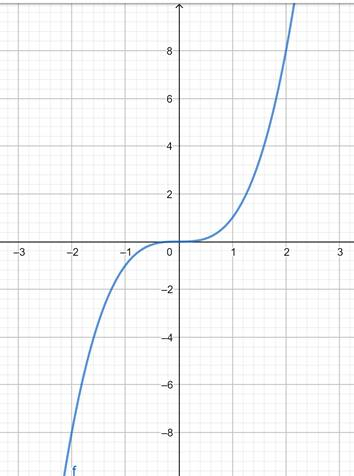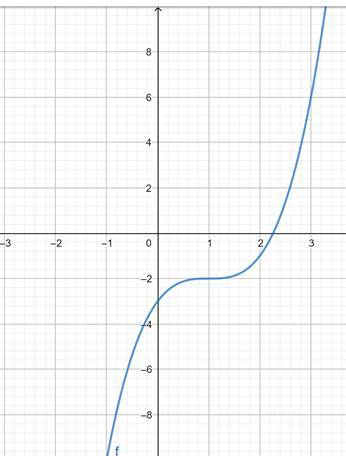# the graph of the function f ( x ) = x 3 .### Precalculus: Mathematics for Calcu...

6th Edition
Stewart + 5 others
Publisher: Cengage Learning
ISBN: 9780840068071### Precalculus: Mathematics for Calcu...

6th Edition
Stewart + 5 others
Publisher: Cengage Learning
ISBN: 9780840068071

#### Solutions

Chapter 2, Problem 6T

a.

To determine

## To sketch : the graph of the function f(x)=x3 .

Expert Solution

### Explanation of Solution

Given information : The function f(x)=x3 is given.

Graph : The graph is given below.Interpretation : The graph of f(x)=x3 is shown above.

b.

To determine

Expert Solution

### Explanation of Solution

Given information : The function g(x)=(x1)32 is given and the graph of f(x)=x3 is given.

Graph : The graph is given below.Interpretation : In this graph, the y intercept shifts to -2 when x = 1, rest all is same.

### Have a homework question?

Subscribe to bartleby learn! Ask subject matter experts 30 homework questions each month. Plus, you’ll have access to millions of step-by-step textbook answers!